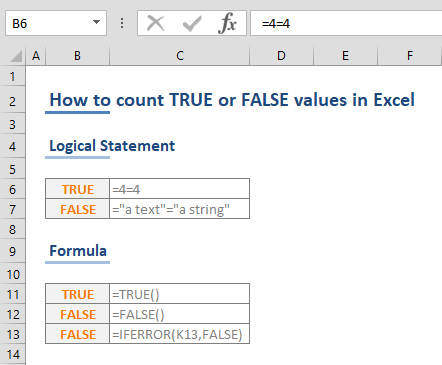TRUE and FALSE are logical (Boolean) values in Excel. They are special values that can be used in formulas as is - although both values looks like a regular text string, you can include them in formulas without the use of double quotes. This behavior may seem confusing, especially when you are trying to count them using functions like COUNTIFS or COUNTIF. In this article, we're going to show you how to count TRUE or FALSE values in Excel.

## TRUE and FALSE Logical Values

First of all, you can generate TRUE and FALSE values as a result of logical statements or a return values from other formulas - thanks to the respective functions for the two: TRUE and FALSE.Both values are displayed as centered text automatically which indicates that these two values are different than standard text strings. Because of this behavior, you need to refer them as special values when using in other formulas.

WRONG: =COUNTIFS(B6:B13,"TRUE")

CORRECT: =COUNTIFS(B6:B13,TRUE)

After this introduction, let's continue with how you can count TRUE or FALSE values in Excel.

## COUNTIFS and COUNTIF Functions

COUNTIFS and COUNTIF functions are used to count values based on a criteria. Both function share a similar syntax, which involves range and criteria pairs. While the newer version, COUNTIFS, can contain more than one pair, the older version only supports a single pair.

We recommend using the COUNTIFS to count TRUE or FALSE values, even if you have a single value to filter. This way you will be utilizing the latest formula, and future-proof your model.

COUNTIFS(criteria_range1, criteria1, [criteria_range2, criteria2], ...)

For each range-criteria pair, first you need to enter the range before the criteria. For example, if you want to search for TRUE values in the range B6:B13, the formula should be

=COUNTIFS(B6:B13,TRUE)

You can add up-to 128 pairs, which means 128 different criteria.You can get the number of FALSE values by replacing TRUE with FALSE. To count the number of FALSE values in the range B6:B13, use the following formula:

=COUNTIFS(B6:B13,FALSE)This example demonstrates how to count TRUE or FALSE values in Excel with the help of COUNTIFS formula. You can learn more about these two formulas from the two related articles:

Function: COUNTIFS

Function: COUNTIF